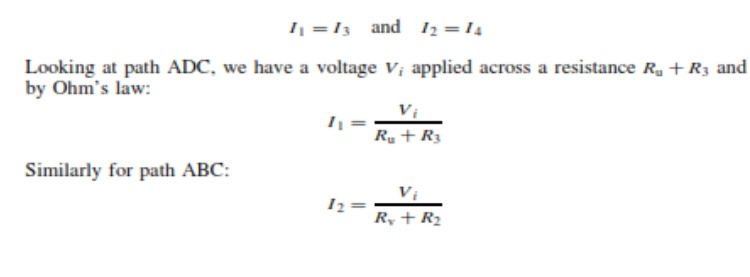Home | | Electrical Engineering and Instrumentation | Wheatstone bridge(Null-type, D.C. bridge)

# Wheatstone bridge(Null-type, D.C. bridge)A null-type bridge with d.c. excitation, commonly known as a Wheatstone bridge, has the form shown in Figure 7.1.

Null-type, d.c. bridge (Wheatstone bridge)

A null-type bridge with d.c. excitation, commonly known as a Wheatstone bridge, has the form shown in Figure 7.1. The four arms of the bridge consist of the unknown resistance Ru, two equal value resistors R2 and R3 and a variable resistor Rv (usually a decade resistance box). A d.c. voltage Vi is applied across the points AC and the resistance Rv is varied until the voltage measured across points BD is zero. This null point is usually measured with a high sensitivity galvanometer.

To analyses the Whetstone bridge, define the current flowing in each arm to be I1 . . . I4 as shown in Figure 7.1. Normally, if a high impedance voltage-measuring instrument is used, the current Im drawn by the measuring instrument will be very small and can be approximated to zero. If this assumption is made, then, for Im D 0:

I1 =I3 and    I2 =I4Study Material, Lecturing Notes, Assignment, Reference, Wiki description explanation, brief detail
Measurements and Instrumentation : Comparison Methods of Measurements : Wheatstone bridge(Null-type, D.C. bridge) |